Search

# Complex mechanics problems using lagrangian mechanics

Updated: Oct 23, 2019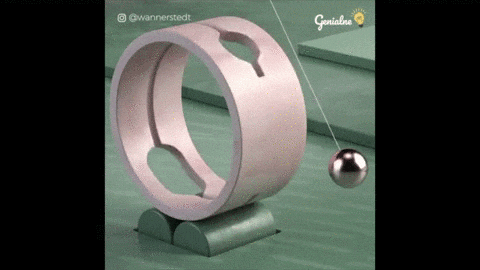To integrate my previous article, in this section we will deal with complex problems that would be extremely hard to solve using newtonian mechanics. We will start with very simple problems and then gradually build up to harder problems.

Of course we won’t solve 100 levelled pendulum kinematics but that’s only because it takes too much time and also there is no point in solving for more than two levels pendulum kinematics since the pattern is obvious.

Problem 1, a simple pendulum :

If you were to solve for a simple pendulum kinematics using old newtonian mechanics, you’ll have to take into account the x and y directions. If not, you’ll have to deal with calculus of variation with vector quantities which is extremely tiring. The advantages of lagrangian mechanics comes in handy in this situation since using the cartesian coordinate system requires a lot of work. In lagrangian mechanics we can use any coordinate system we want as long as the lagrangian could be represented in terms of that preferred coordinate system. Here is an example of a pendulum:The only other possible coordinate system to work with is the cyclic coordinate. Therefore we have to write the lagrangian in terms of theta. Let’s call the length of the wire λ. Using simple trigonometry we see that the generalised form of the y-length of the pendulum is :Which means that the displacement of the mass that’s attached to the pendulum with respect to the ground is equal to :Therefore we can write the potential energy as:Of course we’re assuming that air resistance and friction are negligible. Now for the kinetic energy, the pendulum swings in an arc shaped path, therefore we can rewrite the

kinetic energy of the pendulum in the following form :Where I is the moment of inertia and ω is the angular velocity. And since :Therefore we can conclude that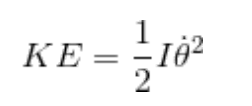Just like that, the lagrangian can be written in the form of theta.Now we can plug the lagrangian into the stationary action equation.Since we’re working with cyclic coordinate system. We can replace q with θ :Plugging in the lagrangian:Differentiating we get :Differentiating again we get :Also:By simplifying we get:And since:therefore: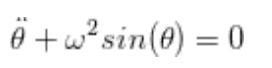Finally we end up with the standard differential equation of an oscillator which is :If you haven’t understood how we got the results from the equation of motion you can check this video about the standard differential equation of oscillators.

Problem 2, a two leveled pendulum :

To increase the complexity, we’ll try to solve for a double pendulum kinematics. Consider the following case :The masses m1 and m2 are attached to each other and thereby to the wall by two non fluid strings, making the two masses swing right and left (also up and down) creating two angles θ1 and θ2. We can denote the positions of the masses by (x1, y1) and (x1, y2) respectively. As you can see the pendulum consists of three nodes. The pivot node, the first mass node and the second mass node. We can consider, the top node or the pivot point, as the starting displacement point of the potential energy. This should make the problem easier to solve because the potential energy of each mass will be expressed in simpler terms. We could also apply this technique to the previous problem problem. As you may have already noticed, the algorithm we go through in lagrangian mechanics is usually something like this :

1. Choose a coordinate system.

2. Solve for the coordinate variable and it's time derivative.

3. Plug the results in the kinetic and potential energy formulas.

4. Get a lagrangian equation.

5. Differentiate.

6. Isolate the wanted kinematic variable on one side of the equation.

As you can see, we have a cyclic coordinate system since we have two angles. Of course we can use the cyclic coordinate system but for the sake of exploration, let’s use the cartesian coordinate system and then switch to the cyclic coordinate system. This should demonstrate another advantage of Lagrangian mechanics. So first we have to have to find the velocity and position of each mass node. The position coordinates of each node mass are :Thereafter we can different with respect to time to get the velocity for the kinetic energy term of the lagrangian. After differentiating with respect to time, we should end up with:Since we have two point masses we could express the potential and kinetic energy of the whole system as the sum of the potential energy of both point masses and the sum of kinetic energy of both point masses respectively. Therefore the kinetic energy of the whole system is :Where the potential energy should be:After some trivial factorisation you should end up with :We can substitute the kinetic energy and the potential energy in the lagrangian formula: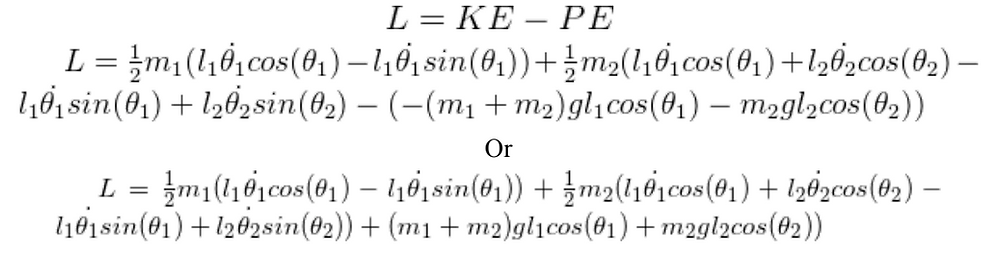Finally, since we have a lagrangian equation. We can obtain the equations of motion of the system by plugging in the lagrangian in the Euler-Lagrange equation. Since we’ve used the cartesian coordinate from the beginning we can switch to the cyclic coordinate because the lagrangian is written in terms of theta. But there are two theta in this situation which theta should we differentiate with respect to ? you might wonder. Well, we will have two equations of motion where we obtain each one by differentiating the lagrangian with respect to each angle. After some trivial differentiating and factorisation we should end up with our first equation of motion :Now, to get the second equation of motion we should differentiate the lagrangian with respect to the second angle (theta two):And finally, after some more trivial differentiating and factorisation we should end up with our second equation of motion :You can manipulate the resultant equation to obtain the term that you’re after. Now, equation may seem boring to some people (even though they shouldn’t), therefore simulations that converts the equation of motion to real observable view may be helpful. Here is a simulation for how a double pendulum in motion looks like :Observation

As you may have already noticed, the lagrangian equation is very long. Consequently it takes time to differentiate. This is a limitation of lagrangian mechanics. Some lagrangian terms can fill up pages. Consider the deconstructed standard model lagrangian equation as an example.If you were to solve this lagrangian formula you can get all the answers to particle physics questions. If you were to rewrite every single term of the equation in its own fundamental terms, you’ll definitely end up with a lagrangian equation that can fill up more than one book. Here, it just becomes humanly impossible to differentiate such lagrangians. No one has solved the equation yet .Therefore, we apply perturbation theory. Unfortunately, we cannot do anything about these long lagrangian equations other than approximating.

Problem 3, A rolling disk that’s attached to a spring

This problem isn’t so complex but it includes a different kind of potential energy which might be helpful to you when encountering different forms of the lagrangian.The equations of motion for this problem should naturally describe the oscillating behaviour for the rolling disk. So to extract the kinetic of the system, we can see that the rolling disk has two kinetic energy values which are the rotational kinetic energy and translational kinetic energy. Therefore, the overall kinetic energy of the system is :Where I is the moment of inertia, omega is the angular velocity, R is the radius of the disk, and v (same thing as x dot) is the velocity of the disk. For the potential energy term, we can use the integral of hooke's law therefore :Now we can just plug this in the lagrangian and plug the lagrangian into the Euler-Lagrange equation. So: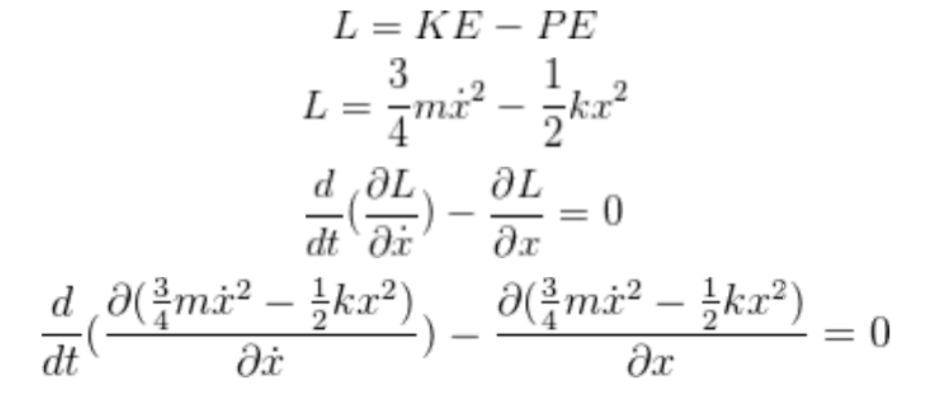After differentiating, we get the equation of motion of the rolling disk :This looks like a simple harmonic equation therefore we can assume that 3k/2 is equal to omega squared and finally, solving the equation gives :this is because x dot dot is a function of time, A is the amplitude of the SMH motion.By expanding omega we get the final equation of the SMH motion :You may not be familiar with this form because the famous equation is :But that form doesn’t include the moment of inertia of the objects that are oscillating. Obviously, lagrangian mechanics gives a better description since it includes more variables.

Problem 4, a pendulum attached to a fulcrum that’s attached to an oscillating spring :

This problem combines the difficulty of the first problem and the third problem. Here’s a labeled diagram to how the problem looks like.We want to find the equation of motion of the mass that’s attached to the end of the pendulum. So let’s start by writing the kinetic energy of the system. Since we don’t know the overall velocity of the mass point, we’ll have to find the velocity in the y-direction and in the x-directions separately. Following the kinetic energy being:After the expansion and the simplification of the velocity terms, you should end up with:Now, for the potential energy term, it consist of two terms. The first one is the potential energy that’s stored in the spring and the second one is the gravitational potential energy, therefore :For the sake of clarification, I have to mention that the gravitational potential energy displacement term starts from the top in this example. That’s why the gravitational potential energy term has a negative value. Finally to write the lagrangian :This problem is special because the lagrangian is dependant on two coordinate system, a cyclic one (comes from the pendulum) and a cartesian one (comes from the spring). Therefore, we’ll have to use two form of the Euler-Lagrange equation, which are:So let’s start with solving the equations. For the sake of space minimisation, I’ll solve each term of the equation as a separate part and then reassemble the resultant terms to form the original Euler-lagrangian equation, I won’t go through the differentiating process because that's both trivial and space/time consuming:Now since we have the four terms that build up the two equations of motion, we can reconstruct the equations to get the wanted equations. Let’s start with solving the cartesian coordinate equation of motion:And finally, the cyclic coordinate equation of motion:As you can see the resultant equations of motion:give you the ability to choose between using a cyclic coordinate or a cartesian coordinate.

Problem 5, A spherical pendulum :

This exercise is fascinating to me, because it demonstrates how easy lagrangian mechanics make real world complex motion dynamics look like. In this problem we’re dealing with three axis and also three angles. So, we’re going to deal with the cartesian coordinate system and later on we’ll switch to the cyclic coordinate.Figure 1, shows a full diagram of what we’re dealing with in this case. x prime, z prime and y prime are the displacement in each axis. Each change in axis create an angle between two lines that go from the new position and the initial position (always 0) and converge at an angle that’s directly perpendicular with the other two axis. This result in three angles that are labeled as phi, theta, phi. This is because the change in the angle in the x direction is equal to the change in the angle in the z direction. Therefore both are given the same variable name. Let’s call the midpoint origo, and the point that the pendulum is attached to asfulcrum. First let’s figure out the kinematics of the system. By definition of the trig functions you shouldbe able to see that :It’s negative because y is positive upward. Based on this we can see that:Since z is parallel with the opposite side of the angle phi that’s created between the axis x and x’. That means that the negative value of that side is equal to the z axis.Now since we have the kinematics in the direction of the three axis. We can get the velocity in each

direction by differentiating the position equations with respect to time.Now, we can start figuring out the kinetic energy of the pendulum by plugging in cartesian coordinate

variables and thereafter converting to the cyclic coordinate variables.After expanding and simplifying the velocity terms, you should end up with :Thanks to the properties of the trig functions we can simplify this even more. Since cosine squared theta multiplied by sine squared theta is equal to one. Therefore the resultant form of the kinetic energy term is:It’s pretty easy to obtain the potential energy term since it only depends on the height or in other words the y coordinate.Now that we have both the kinetic and potential energy terms, we can finally write the lagrangian form.Last but not least, we can plug in the lagrangian into the euler-lagrange equation to obtain the equation of motion.Dividing by ml squared on both sides we get the first equation of motion :Since this is a two degree of freedom, there must be another equation of motion which is:Obviously the ml squared cancels out, surprisingly, resulting in a conserved value:Dividing by sine squared theta, we get the second equation of motion :